• 栈式自编码神经网络(Stacked Autoencoder,SA)，是对自编码网络的一种使用方法，是一个由多层训练好的自编码器组成的神经网络。由于网络中的每一层都是单独训练而来，相当于都初始化了一个合理的数值。所以，这样的...
介绍
栈式自编码神经网络(Stacked Autoencoder,SA)，是对自编码网络的一种使用方法，是一个由多层训练好的自编码器组成的神经网络。由于网络中的每一层都是单独训练而来，相当于都初始化了一个合理的数值。所以，这样的网络会更容易训练，并且有更快的收敛性及更好的准确度。
栈式自编码常常被用于预训练（初始化）神经网络之前的权重预训练步骤。例如，在一个分类问题上，可以按照从前向后的顺序执行每一层通过自编码器来训练，最终将网络中最深层的输出作为softmax分类器的输入特征，通过softmax层将其分开。
下面以训练一个包含两个隐含层的展示自编码网络为例子。
（1）训练一个自编码器，得到原始输入的一阶特征表示h(1)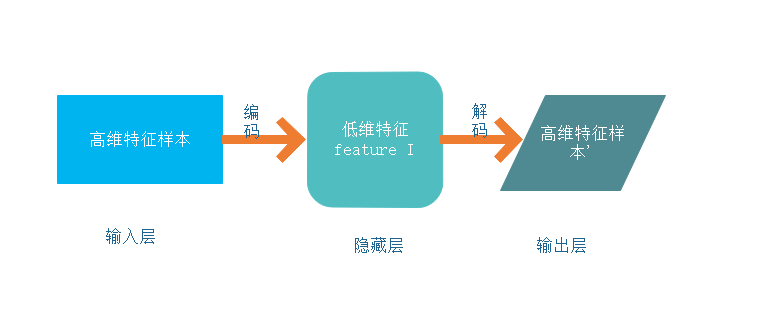（2）将上一步输出的特征h(1)作为输入，对其进行再一次的自编码，并同时获取特征h(2)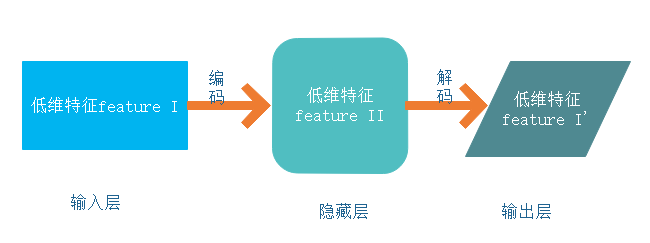(3)把上一步的特征h(2)连接上softmax分类器，得到一个图片数字标签分类的模型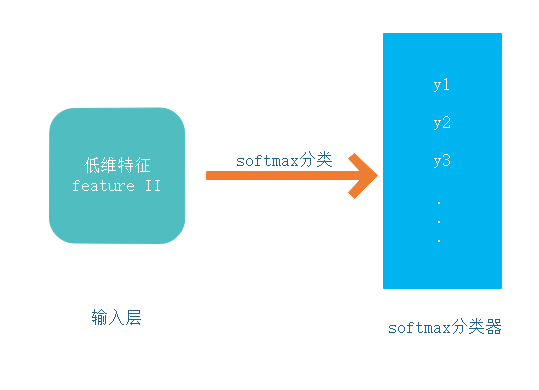栈式自编码在深度学习中的意义
SA的优点如下：
1.每一层都可以单独训练，保证降维特征的可控性
2.对于高维度的分类问题，一下子拿出一套完整可用的模型相对来说并不是容易的事情，因为节点太多，参数热爱多，一味地增加深度只会使结果越来越不可控，成为彻底的黑盒，而使用栈式自编码逐层降维，可以将复杂问题简单化，更容易完成任务。
3.任意深层，理论上是越深层的神经网络对现实的拟合度越高，但是传统的多层神经网络，由于使用的是误差反向传播方式，导致层越深，传播的误差越小。栈式自编码巧妙地绕过这个问题，直接使用降维后的特征值进行二次训练，可以任意层数的加深。
参考资料
《深度学习之Tensorflow 入门、原理与进阶实战》 李金洪


展开全文•研究论文
• 部分内容来自：http://ufldl.stanford.edu/wiki/index.php/%E6%A0%88%E5%BC%8F%E8%87%AA%E7%BC%96%E7%A0%81%E7%AE%97%E6%B3%95栈式自编码...对于一个层栈式自编码的编码过程就是，按照从前向后的顺序执行每一层自编...
部分内容来自：http://ufldl.stanford.edu/wiki/index.php/%E6%A0%88%E5%BC%8F%E8%87%AA%E7%BC%96%E7%A0%81%E7%AE%97%E6%B3%95栈式自编码神经网络是一个由多层稀疏自编码器组成的神经网络，其前一层自编码器的输出作为其后一层自编码器的输入。对于一个层栈式自编码的编码过程就是，按照从前向后的顺序执行每一层自编码器的编码步骤。自编码器的隐含层t会作为t+1层的输入层，第一个输入层就是整个神经网络的输入层，利用贪心算法训练每一层的步骤如下：1)首先采用稀疏自编码网络，先训练从输入层到H1层的参数：训练完毕后，我们去除解码层，只留下从输入层到隐藏层的编码阶段。2)接着我们训练从H1到H2的参数我们把无标签数据的H1层神经元的激活值，作为H2层的输入层，然后在进行自编码训练：最后训练完毕后，再去除H2层的解码层。3)对所有层重复1)和2)，即移除前面自编码器的输出层，用另一个自编码器代替，再用反向传播进行训练。4)以上步骤称为预训练，但是通过这个训练并没有得到一个从输入数据到输出标记的映射，通常的办法是在网络的最后一层增加一个或多个连接层，整个网络可以看做是一个多层感知机，并用反向传播的方式进行训练，这一步也被称为微调。
展开全文• 去噪自编码和栈式自编码讲解及代码 https://www.cnblogs.com/zyly/p/9078786.html
去噪自编码和栈式自编码讲解及代码
https://www.cnblogs.com/zyly/p/9078786.html


展开全文• 栈式自编码神经网络（Stacked Autoencoder, SA），是对自编码网络的一种使用方法，是一个由多层训练好的自编码器组成的神经网络。由于网络中的每一层都是单独训练而来，相当于都初始化了一个合理的数值。所以，这样...
       栈式自编码神经网络（Stacked Autoencoder, SA），是对自编码网络的一种使用方法，是一个由多层训练好的自编码器组成的神经网络。由于网络中的每一层都是单独训练而来，相当于都初始化了一个合理的数值。所以，这样的网络会更容易训练，并且有更快的收敛性及更高的准确度。

栈式自编码常常被用于预训练（初始化）深度神经网络之前的权重预训练步骤。例如在一个分类问题上，可以按照从前向后的顺序执行每一层通过自编码器来训练，最终将网络中最深层的输出作为softmax分类器的输入特征，通过softmax层将其分开。

为了使这个过程容易理解，下面以训练一个包含两个隐含层的栈式自编码网络为例。

（1）训练一个自编码器，得到原始输入的一阶特征表示h。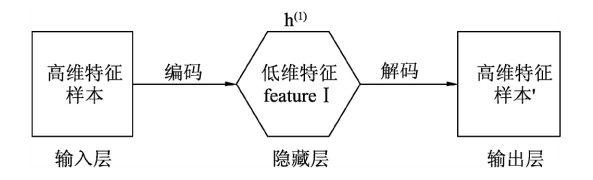（2）将上一步输出的特征h作为输入，对其进行再一次的自编码，并同时获取特征h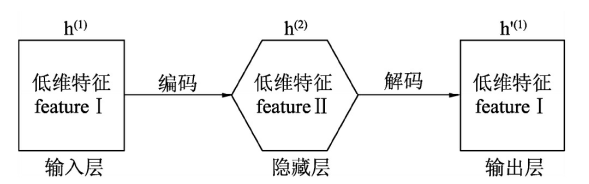（3）把上一步的特征h连上softmax分类器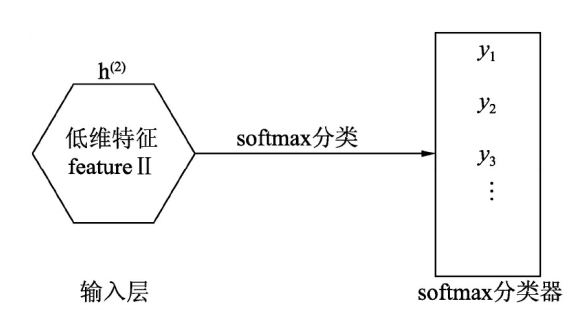（4）把这3层结合起来，就构成了一个包含两个隐藏层加一个softmax的栈式自编码网络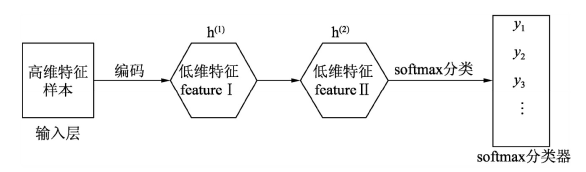常用方法：代替和级联。

实例：首先建立一个去噪自编码，然后再对第一层的输出做一次简单的自编码压缩，然后再将第二层的输出做一个softmax的分类，最后，把这3个网络里的中间层拿出来，组成一个新的网络进行微调。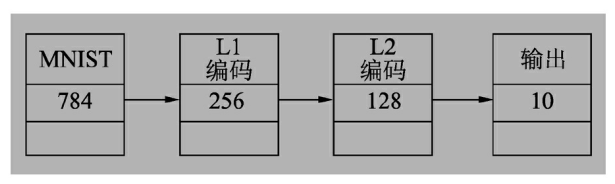import numpy as np
import tensorflow as tf
import matplotlib.pyplot as plt
from tensorflow.examples.tutorials.mnist import input_data

train_X   = mnist.train.images
train_Y = mnist.train.labels
test_X    = mnist.test.images
test_Y  = mnist.test.labels

tf.reset_default_graph()
# 参数
n_input    = 784
n_hidden_1 = 256 #第一层自编码
n_hidden_2 = 128 #第二层自编码
n_classes = 10

# 第一层
x = tf.placeholder("float", [None, n_input])
y = tf.placeholder("float", [None, n_input])
dropout_keep_prob = tf.placeholder("float")
# 第二层
l2x = tf.placeholder("float", [None, n_hidden_1])
l2y = tf.placeholder("float", [None, n_hidden_1])
# 第三层
l3x = tf.placeholder("float", [None, n_hidden_2])
l3y = tf.placeholder("float", [None, n_classes])

# WEIGHTS
weights = {
#网络1  784-256-784
'h1': tf.Variable(tf.random_normal([n_input, n_hidden_1])),
'l1_h2': tf.Variable(tf.random_normal([n_hidden_1, n_hidden_1])),
'l1_out': tf.Variable(tf.random_normal([n_hidden_1, n_input])),
#网络2  256-128-256
'l2_h1': tf.Variable(tf.random_normal([n_hidden_1, n_hidden_2])),
'l2_h2': tf.Variable(tf.random_normal([n_hidden_2, n_hidden_2])),
'l2_out': tf.Variable(tf.random_normal([n_hidden_2, n_hidden_1])),
#网络3  128-10
'out': tf.Variable(tf.random_normal([n_hidden_2, n_classes]))
}
biases = {
'b1': tf.Variable(tf.zeros([n_hidden_1])),
'l1_b2': tf.Variable(tf.zeros([n_hidden_1])),
'l1_out': tf.Variable(tf.zeros([n_input])),

'l2_b1': tf.Variable(tf.zeros([n_hidden_2])),
'l2_b2': tf.Variable(tf.zeros([n_hidden_2])),
'l2_out': tf.Variable(tf.zeros([n_hidden_1])),

'out': tf.Variable(tf.zeros([n_classes]))
}
#第一层的编码输出
#l1 编码
def noise_l1_autodecoder(layer_1, _weights, _biases, _keep_prob):
layer_1out = tf.nn.dropout(layer_1, _keep_prob)
layer_2out = tf.nn.dropout(layer_2, _keep_prob)
return tf.nn.sigmoid(tf.matmul(layer_2out, _weights['l1_out']) + _biases['l1_out'])
# 第一层的解码输出
l1_reconstruction = noise_l1_autodecoder(l1_out, weights, biases, dropout_keep_prob)
# 计算损失
l1_cost = tf.reduce_mean(tf.pow(l1_reconstruction-y, 2))
#第二层的编码输出
def l2_autodecoder(layer1_2, _weights, _biases):
return tf.nn.sigmoid(tf.matmul(layer1_2out, _weights['l2_out']) + _biases['l2_out'])
# 第二层的解码输出
l2_reconstruction = l2_autodecoder(l2_out, weights, biases)
l2_cost = tf.reduce_mean(tf.pow(l2_reconstruction-l2y, 2))
#l3  分类
l3_out = tf.matmul(l3x, weights['out']) + biases['out']
l3_cost = tf.reduce_mean(tf.nn.softmax_cross_entropy_with_logits(logits=l3_out, labels=l3y))
#3层 级联
#1联2
# 2联3
pred = tf.matmul(l1_l2out, weights['out']) + biases['out']
cost3 = tf.reduce_mean(tf.nn.softmax_cross_entropy_with_logits(logits=pred, labels=l3y))
print ("l3 级联 ")
# 训练
epochs     = 50
batch_size = 100
disp_step  = 10
load_epoch =49

第一层训练

# 第一层训练
with tf.Session() as sess:
sess.run(tf.global_variables_initializer())
print ("开始训练")
for epoch in range(epochs):
num_batch  = int(mnist.train.num_examples/batch_size)
total_cost = 0.
for i in range(num_batch):
batch_xs, batch_ys = mnist.train.next_batch(batch_size)
batch_xs_noisy = batch_xs + 0.3*np.random.randn(batch_size, 784)
feeds = {x: batch_xs_noisy, y: batch_xs, dropout_keep_prob: 0.5}
sess.run(l1_optm, feed_dict=feeds)
total_cost += sess.run(l1_cost, feed_dict=feeds)
# DISPLAY
if epoch % disp_step == 0:
print ("Epoch %02d/%02d average cost: %.6f"
% (epoch, epochs, total_cost/num_batch))
print(sess.run(weights['h1']))
print (weights['h1'].name)
print ("完成")
show_num = 10
test_noisy = mnist.test.images[:show_num] + 0.3*np.random.randn(show_num, 784)
encode_decode = sess.run(
l1_reconstruction, feed_dict={x: test_noisy, dropout_keep_prob: 1.})
f, a = plt.subplots(3, 10, figsize=(10, 3))
for i in range(show_num):
a[i].imshow(np.reshape(test_noisy[i], (28, 28)))
a[i].imshow(np.reshape(mnist.test.images[i], (28, 28)))
a[i].matshow(np.reshape(encode_decode[i], (28, 28)), cmap=plt.get_cmap('gray'))
plt.show()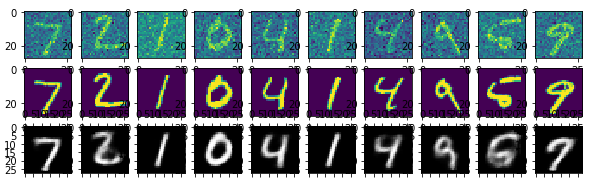第二层训练

# 第二层训练
with tf.Session() as sess:
sess.run(tf.global_variables_initializer())
print ("开始训练")
for epoch in range(epochs):
num_batch  = int(mnist.train.num_examples/batch_size)
total_cost = 0.
for i in range(num_batch):
batch_xs, batch_ys = mnist.train.next_batch(batch_size)

l1_h = sess.run(l1_out, feed_dict={x: batch_xs, y: batch_xs, dropout_keep_prob: 1.})
_,l2cost = sess.run([optm2,l2_cost], feed_dict={l2x: l1_h, l2y: l1_h })
total_cost += l2cost

# log
if epoch % disp_step == 0:
print ("Epoch %02d/%02d average cost: %.6f"
% (epoch, epochs, total_cost/num_batch))
print(sess.run(weights['h1']))
print (weights['h1'].name)
print ("完成  layer_2 训练")
show_num = 10
testvec = mnist.test.images[:show_num]
out1vec = sess.run(l1_out, feed_dict={x: testvec,y: testvec, dropout_keep_prob: 1.})
out2vec = sess.run(l2_reconstruction, feed_dict={l2x: out1vec})
f, a = plt.subplots(3, 10, figsize=(10, 3))
for i in range(show_num):
a[i].imshow(np.reshape(testvec[i], (28, 28)))
a[i].matshow(np.reshape(out1vec[i], (16, 16)), cmap=plt.get_cmap('gray'))
a[i].matshow(np.reshape(out2vec[i], (16, 16)), cmap=plt.get_cmap('gray'))
plt.show()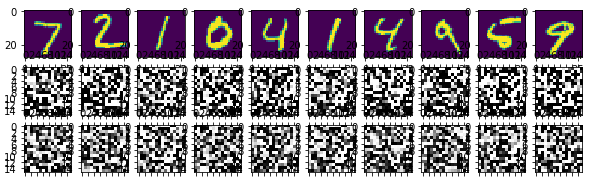第三层训练

# 第三层
with tf.Session() as sess:
sess.run(tf.global_variables_initializer())
print ("开始训练")
for epoch in range(epochs):
num_batch  = int(mnist.train.num_examples/batch_size)
total_cost = 0.
for i in range(num_batch):
batch_xs, batch_ys = mnist.train.next_batch(batch_size)
l1_h = sess.run(l1_out, feed_dict={x: batch_xs, y: batch_xs, dropout_keep_prob: 1.})
l2_h = sess.run(l2_out, feed_dict={l2x: l1_h, l2y: l1_h })
_,l3cost = sess.run([l3_optm,l3_cost], feed_dict={l3x: l2_h, l3y: batch_ys})
total_cost += l3cost
# DISPLAY
if epoch % disp_step == 0:
print ("Epoch %02d/%02d average cost: %.6f"
% (epoch, epochs, total_cost/num_batch))
print ("完成  layer_3 训练")
# 测试 model
correct_prediction = tf.equal(tf.argmax(pred, 1), tf.argmax(l3y, 1))
# 计算准确率
accuracy = tf.reduce_mean(tf.cast(correct_prediction, "float"))
print ("Accuracy:", accuracy.eval({x: mnist.test.images, l3y: mnist.test.labels}))

级联微调

# 级联微调
with tf.Session() as sess:
sess.run(tf.global_variables_initializer())
print ("开始训练")
for epoch in range(epochs):
num_batch  = int(mnist.train.num_examples/batch_size)
total_cost = 0.
for i in range(num_batch):
batch_xs, batch_ys = mnist.train.next_batch(batch_size)
feeds = {x: batch_xs, l3y: batch_ys}
sess.run(optm3, feed_dict=feeds)
total_cost += sess.run(cost3, feed_dict=feeds)
# DISPLAY
if epoch % disp_step == 0:
print ("Epoch %02d/%02d average cost: %.6f"
% (epoch, epochs, total_cost/num_batch))
print ("完成  级联 训练")
# 测试 model
correct_prediction = tf.equal(tf.argmax(pred, 1), tf.argmax(l3y, 1))
# 计算准确率
accuracy = tf.reduce_mean(tf.cast(correct_prediction, "float"))
print ("Accuracy:", accuracy.eval({x: mnist.test.images, l3y: mnist.test.labels}))

开始训练
Epoch 00/50 average cost: 1.544741
Epoch 10/50 average cost: 0.070898
Epoch 20/50 average cost: 0.010157
Epoch 30/50 average cost: 0.001123
Epoch 40/50 average cost: 0.000119
完成  级联 训练
Accuracy: 0.9613

可以看到，由于网络模型中各层的初始值已经训练好了，所以开始就是很低的错误率。


展开全文• ## 栈式自编码算法

千次阅读 2016-05-01 11:01:05
栈式自编码算法 Contents  [hide] 1 概述2 训练3 具体实例4 讨论5 中英文对照6 中文译者 概述 逐层贪婪训练法依次训练网络的每一层，进而预训练整个深度神经网络。在本...神经网络 深度学习 机器学习
• 基于tensoflow1.8实现的栈式自动编码器，共4层，具体为输入层，降维层1，降维层2，分类层（softmax）
• UFLDL Exercise: Stacked autoencoder（栈式自编码算法）matlab实验代码 可以直接运行
• 针对传统机器学习方法不能有效地提取恶意代码的潜在特征，提出了基于栈式自编码（stacked auto encoder，SAE）的恶意代码分类算法。其次从大量训练样本中学习并提取恶意代码纹理图像特征、指令语句中的隐含特征；在...恶意代码 分类
• 栈式自编码神经网络（Stacked Autoencoder, SA）是对自编码网络的一种使用方法。而前面说的自编码（包括卷积，变分，条件变分都只是一种自编码结构），而这里是应用。 SA 是一个由多层训练好的自编码器组成的神经...
• 从自编码到栈式自编码 原文地址：http://blog.csdn.NET/hjimce/article/details/49106869 作者：hjimce 一、什么是自编码  简单的自编码是一种三层神经网络模型，包含了数据输入层、隐藏层、输出重构层，同时...
• 根据所解决的问题不同 ，自编码可以有许多种不同形式的变形，例如： 去噪自编码器(DAE)、变分自编码器 (VAE)、收缩自编码器(CAE)和稀疏自编码器等 。下面我们先从去噪自编码讲起。一 去噪自编码要想取得好的特征只...
• 栈式自编码算法 Contents [hide] 1 概述2 训练3 具体实例4 讨论5 中英文对照6 中文译者 概述 逐层贪婪训练法依次训练网络的每一层，进而预训练整个深度神经网络。在本节中，我们将会学习如何将自编码器...Stanford
• 从自编码到栈式自编码 原文地址：http://blog.csdn.NET/hjimce/article/details/49106869 作者：hjimce 一、什么是自编码  简单的自编码是一种三层神经网络模型，包含了数据输入层、隐藏层、输出重构层，同时...
• 2.对于高维度的分类问题，因为节点太多，参数太多，一味地增加深度只会使结果越来越不可控，成为彻底的黑盒，而使用栈式自编码逐层降维，可以将复杂问题简单化，更容易完成任务。 3.任意深层，理论上是越深层的神经...TensorFlow 深度学习
• ## 基于tensorflow的栈式自编码器实现

万次阅读 热门讨论 2017-03-30 11:37:36
这周完全没有想法要看栈式编码器的，谁知误入桃花源，就暂且把栈式自编码器看了吧。由于手上有很多数值型的数据，仅仅是数据，没有标签，所以，迫切需要通过聚类抽出特征。无意间看到别人家公司的推荐系统里面用到...tensorflow
• 上期我们一起学习了深度学习中的自编码器的相关知识，深度学习算法(第26期)----深度网络中的自编码器今天我们更进一步一起学一下栈式自编码器。栈式自编码器跟之前我们学过的其他神经网络一样...
• 1.单层编码能够尽量保留输入层的信息，如果在第一层编码的基础上继续构建一层自动编码器，那么新的编码能够尽量保留第一层编码的信息，也就能保留输入的绝大部分信息，这种叠加的自动编码器称为栈式自动编码器。...深度学习 机器学习 深度信念网络
• 栈式自动编码器(Stacked AutoEncoder) 起源：自动编码器 单自动编码器，充其量也就是个强化补丁版PCA，只用一次好不过瘾。 于是Bengio等人在2007年的 Greedy Layer-Wise Training of Deep Networks中， 仿照...
• 最近研究了一篇论文，关于检测DDos攻击，使用了深度学习中 栈式自编码的算法，现在简要介绍一下内容论文下载 讨论班讲解pdf-by airghc ppt DDOS: Distributed Denial of Service(分布式拒绝服务)Purpose:...
• 自编码器及其变形很多，本篇博客目前主要基于普通自编码器、欠完备自编码器、稀疏自编码器和去噪自编码器，会提供理论+实践（有的理论本人没有完全理解，就先没有写上，后更）。另外，关于收缩自编码器、变分自编码...
• https://blog.csdn.net/hjimce/article/details/49106869
• 之前介绍了AutoEncoder及其几种拓展结构，如DAE，CAE等，本篇博客介绍栈式自编码器。 模型介绍 普通的AE模型通过多层编码解码过程，得到输出，最小化输入输出的差异从而使模型学到有用的特征。但是这种AE结构又一个...深度学习
• 栈式自编码器(stacked autoencoder, SA)   即多个自编码器堆叠而成，是深度神经网络中的一种。   例如：多个去噪自编码器的堆叠就变成了stacked denoised autoencoder (SDA)。 优点和缺点   stacked denoised ......